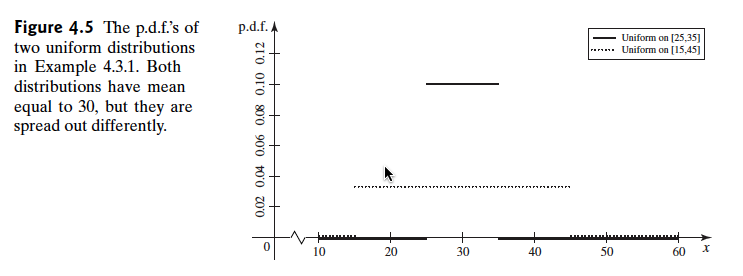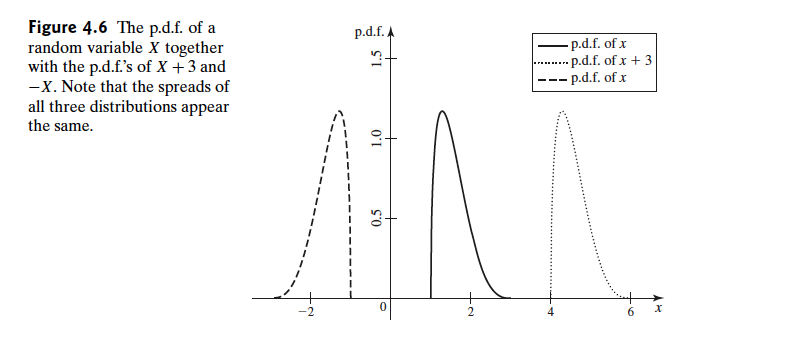Abstract: 本文介绍继期望之后分布的另一个重要数学性质，方差
Keywords: Variance,Standard Deviation

# 方差## 方差和标准差的定义 Definitions of the Variance and the Standard Deviation

Definition Variance/Standard Deviation.Let $X$ be a random variable with finite mean $\mu=E(X)$ ,The variance of $X$ denoted by $Var(x)$ ,is defined as follows:
$$Var(X)=E[(X-\mu)^2]$$

$$Var(A)=\int^{35}_{25}(a-30)^2\frac{1}{10}da=\frac{1}{10}\frac{x^3}{3}\arrowvert^5_{x=-5}=\frac{25}{3}$$

Theorem Alternative Method for Calculating the Variance.For every random variable $X$ , $Var(X)=E(X^2)-[E(X)]^2$

\begin{aligned} Var(X) & =E[(X-\mu)^2]\\ & =E(X^2-2\mu X+\mu^2)\\ & =E(X^2)-2\mu E(X)+ \mu^2\\ & =E(X^2)-\mu^2 \end{aligned}
Q.E.D

## 方差的性质 Properties of the Variance

Theorem For each $X$ , $Var(X)\geq 0$ .If $X$ is a bounded random varibale,then $Var(X)$ must exist and be finite.

Theorem $Var(X=0)$ if and only if there exists a constant c such that $Pr(X=c)=1$ ,then $\mu=c$ and $Pr[(X-c)^2=0]=1$

1. 假设存在一个随机变量 $X$ 和一个常数c 满足 $Pr(X=c)=1$ 那么 $E(X)=c$ 并且 $Pr[(X-c)^2=0]=1$ 然后就有了
$$Var(X)=E[(X-c)^2]=0$$

2. 反过来假设 $Var(X)=0$

• 那么就有 $Pr[(X-\mu)^2\geq 0]=1$ 。
• 但是又因为 $E[(X-\mu)^2]=0$ 也就是 $0=\int^{\infty}_{-\infty}Pr【(x-\mu)^2】(x-\mu)^2dx$
• 根据定理（ Theorem Suppose that $E(x)=a$ and that either $Pr(X\geq a)=1$ or $Pr(X\leq a)=1$ Then $Pr(X=a)=1$）
• 可以得到
$$Pr[(X-\mu)^2=0]=1$$
证毕(其实2中用的那个定理有点问题，我还没想明白)

Theorem For constant $a$ and $b$ let $Y=aX+b$ Then
$$Var(Y)=a^2Var(X)$$
and $\sigma_Y=|a|\sigma_X$

\begin{aligned} Var(Y) & =E[(aX+b-a\mu-b)^2]=E[(aX-a\mu)^2]\\ & =a^2E[(X-\mu)^2]=a^2Var(X) \end{aligned}$$Var(-x)=Var(x)$$

Theorem If $X_1,\dots,X_n$ are independent random variable with finite means,Then
$$Var(X_1+\dots +X_n)=Var(X_1)+\dots+Var(X_n)$$

$$E[X_1]+E[X_2]=\mu_1+\mu_2$$

\begin {aligned} Var(X_1+X_2)& =E[(X_1+X_2-\mu_1-\mu_2)^2]\\ &=E[(X_1-\mu_1)^2+(X_2-\mu_2)^2+2(X_1-\mu_1)(X_2-\mu_2)]\\ &=E[(X_1-\mu_1)^2]+E[(X_2-\mu_2)^2]+E[2(X_1-\mu_1)(X_2-\mu_2)]\\ &=Var(X_1)+Var(X_2)+E[2(X_1-\mu_1)(X_2-\mu_2)] \end{aligned}

$$\text{When } X_1 \text{ and } X_2 \text{ are independent}\\ E[2(X_1-\mu_1)(X_2-\mu_2)]=2\times E[(X_1-\mu_1)]E[(X_2-\mu_2)]=0$$

Corollary If $X_1,\dots,X_n$ are independent random varibales with finite means,and if $a_1,\dots,a_n$ then
$$Var(a_1X_1+\dots+a_nX_n)=a^2Var(X_1)+\dots+a_n^2Var(X_n)$$

## 二项分布的方差 The Variance of a Binomial Distribution

$$Var(X)=\sum^{n}_{i=1}Var(X_i)$$

$$Var(X_i)=E(X_i^2)-[E(X_i)]^2=p-p^2$$

\begin{aligned} Var(X) & =\sum^{n}_{i=1} Var(X_i)\\ & =\sum^{n}_{i=1}(p-p^2)\\ &=np(1-p) \end{aligned}

## 四分位数范围 Interquartile Range

Definition Interquartile Range(IQR). Let X be a random varibale with quatile function $F^{-1}(p)$ for $0<p<1$ .The interquartile range (IQR) is defined to be $F^{-1}(0.75)-F^{-1}(0.25)$

0%